Courses

Test: Graph Theory

10 Questions MCQ Test Network Theory (Electric Circuits) | Test: Graph Theory

Description
Attempt Test: Graph Theory | 10 questions in 30 minutes | Mock test for Electrical Engineering (EE) preparation | Free important questions MCQ to study Network Theory (Electric Circuits) for Electrical Engineering (EE) Exam | Download free PDF with solutions
QUESTION: 1

Consider the following circuits :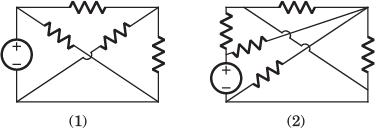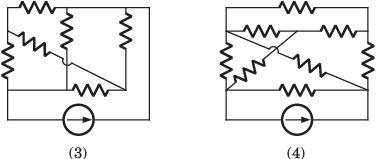The planner circuits are

Solution:

The circuit 1 and 2 are redrawn as below. 3 and 4 can not be redrawn on a plane without crossing other branch.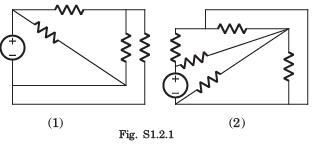QUESTION: 2

Consider the following graphs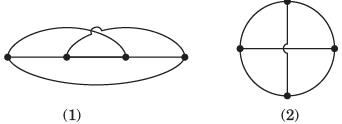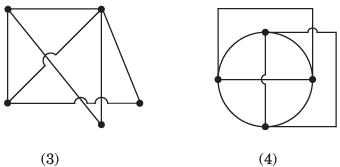Non-planner graphs are

Solution:

Other three circuits can be drawn on plane without crossing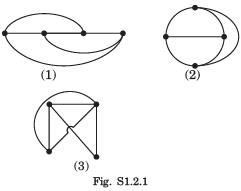QUESTION: 3

A graph of an electrical network has 4 nodes and 7 branches. The number of links l, with respect to the chosen tree, would be

Solution:

l = b - ( n - 1) = 4.

QUESTION: 4

For the graph shown in fig. P.1.1.4 correct set is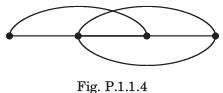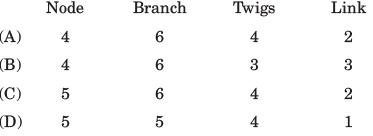Solution:

There are 4 node and 6 branches.
t = n - 1 = 3, l = b - n + 1 = 3

QUESTION: 5

A tree of the graph shown in fig. P.1.2.5 is​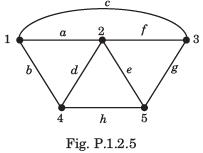Solution:

From fig. it can be seen that afhg is a tree of given graph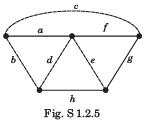QUESTION: 6

Branch current and loop current relation are expressed in matrix form as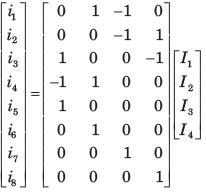where irepresent branch current and Ik loop current. The number of independent node equation are

Solution:

Number of branch = 8
Number of twigs = 8 - 4 = 4
Number of twigs =number of independent node equation.

QUESTION: 7

If the number of branch in a network is b, the number of nodes is n and the number of dependent loop is l, then the number of independent node equations will be

Solution:

The number of independent node equation are n - 1.

QUESTION: 8

Branch current and loop current relation are expressed in matrix form as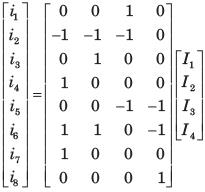where ij represent branch current and Ik loop current.
Q. The rank of incidence matrix is

Solution:

Number of branch b=8
Number of link l = 4
Number of twigs t = b - l = 4
rank of matrix = n - 1 = t = 4

QUESTION: 9

Branch current and loop current relation are expressed in matrix form aswhere ij represent branch current and Ik loop current.
Q. The directed graph will be

Solution:

We know the branch current and loop current are related as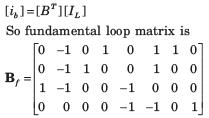f-loop 1 include branch (2, 4, 6, 7) and direction of branch–2 is opposite to other (B only).

QUESTION: 10

A network has 8 nodes and 5 independent loops.The number of branches in the network is

Solution:

l = b - ( n - 1)
⇒ 5= b - 7, b = 12Use Code STAYHOME200 and get INR 200 additional OFF Use Coupon Code

Track your progress, build streaks, highlight & save important lessons and more!

Similar ContentRelated tests